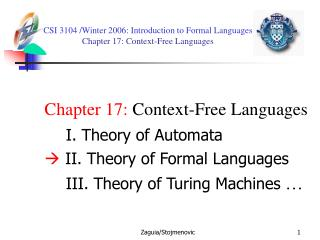Download PresentationCSI 3104 /Winter 2006 : Introduction to Formal Languages Chapter 17: Context-Free Languages

# CSI 3104 /Winter 2006 : Introduction to Formal Languages Chapter 17: Context-Free Languages - PowerPoint PPT PresentationDownload Presentation## CSI 3104 /Winter 2006 : Introduction to Formal Languages Chapter 17: Context-Free Languages

- - - - - - - - - - - - - - - - - - - - - - - - - - - E N D - - - - - - - - - - - - - - - - - - - - - - - - - - -
##### Presentation Transcript

1. CSI 3104 /Winter 2006: Introduction to Formal Languages Chapter 17: Context-Free Languages Chapter 17: Context-Free Languages I. Theory of Automata II. Theory of Formal Languages III. Theory of Turing Machines … Zaguia/Stojmenovic

2. Chapter 17: Context-Free Languages • Theorem. The set of context-free languages is closed under union, concatenation, and Kleene closure. • Union. L1+L2 L1 and L2 are generated by two context-free grammars G1 and G2. We replace each nonterminal X in G1 by X1, and each nonterminal X in G2 by X2. We add the productions: S  S1 S  S2 L1+L2 is the language generated by this new CFG. Zaguia/Stojmenovic

3. Chapter 17: Context-Free Languages Example: L1 = PALINDROME: S  aSa | bSb | a | b | Λ L2 = anbn: S  aSb | Λ L1+L2 :S  S1 | S2 S1 aS1a | bS1b | a | b | Λ S2 aS2b | Λ Example:L1 = {aa,bb}: S  aA | bB A  a B  b L2 = {Λ}: S Λ L1+L2 :S  S1 | S2 S1 aA1 | bB1 A1 a B1 b S2Λ Zaguia/Stojmenovic

4. START START START ACCEPT ACCEPT ACCEPT Chapter 17: Context-Free Languages Proof by machines Zaguia/Stojmenovic

5. START a START READ READ POP ACCEPT a a a PUSH a ACCEPT b PUSH b Chapter 17: Context-Free Languages (begin with a) (contain aa) Zaguia/Stojmenovic

6. READ READ POP START a a PUSH a a a ACCEPT b PUSH b Chapter 17: Context-Free Languages L1 + L2 Zaguia/Stojmenovic

7. Chapter 17: Context-Free Languages • Concatenation. L1L2 Similar to union except we add: S  S1S2 Example: L1= palindrome: S  aSa | bSb | a | b |  L2= {anbn}: S  aSb |  L1L2: S  S1S2 S1 aS1a | bS1b | a | b |  S2 aS2b |  proof by PDAs? Zaguia/Stojmenovic

8. L* • Kleene star. L* We replace S by S1 and add: S  S1S | Λ S  S1S  S1S1S  S1S1S1S  … Example: L: S  aSa | bSb | a | b |  • L*: S S1S |  • S1 aS1a | bS1b | a | b |  Zaguia/Stojmenovic

9. Chapter 17: Context-Free Languages • Theorem. The intersection of two context-free languages may or may not be context-free. • L1 = anbnam S  XA X  aXb / ab A  aA / a • L2 = anbmam S  AX X  bXa / ba A  aA / a • L1 ∩ L2 = anbnan (is not a context-free language ) Zaguia/Stojmenovic

10. Chapter 17: Context-Free Languages • Theorem. The intersection of a context-free language and a regular language is always context-free. Proof: By constructive algorithm using pushdown automata, similar to the intersection algorithm for two finite automata. (details not covered) Zaguia/Stojmenovic

11. Example: use the theorem • L= doubleword = {ww} = {abbabb,…} • Assume L is CFL • L ∩ {a+b+a+b+} = {anbmanbm} • is then context free (∩ regular lang.) • But we have proven that {anbmanbm} is not CFL •  contradiction. Zaguia/Stojmenovic

12. Chapter 17: Context-Free Languages • Theorem. The complement of a context-free language may or may not be context-free. When the PDA is deterministic with other properties, we could use for the complement a similar technique as for FA Zaguia/Stojmenovic

13. START a a X a READ READ POP POP POP PUSH a X b b,L D D PUSH b REJECT a,b REJECT a,L b b D ACCEPT Chapter 17: Context-Free Languages PALINDROME-X Zaguia/Stojmenovic

14. START a a X a PUSH a READ READ POP POP POP X b b,L D D PUSH b ACCEPT a,b ACCEPT a,L b b D REJECT Chapter 17: Context-Free Languages For the complement: We interchange the states ACCEPT and REJECT. It does not work all the time. Zaguia/Stojmenovic

15. Complement of a CFL may not be a CFL • Proof by indirect arguments • Suppose complement of every CFL is a CFL. • L1 and L2 CFLs  L1’ and L2’ CFLs  L1’+L2’ CFLs  (L1’+L2’)’ = L1  L2 is CFL • Contradicts intersection theorem. Zaguia/Stojmenovic

16. Nondeterminism • L accepts w if some paths lead to accept (some paths may lead to reject) • L rejects w if all paths lead to reject • Exchange accept and reject states: • L’ rejects w if some paths lead to reject ?? • L’ accepts w if all paths lead to accept ?? • Nondeterminism does not support complement Zaguia/Stojmenovic

17. Example • L’={anbnan} is not CFL but L is CFL • L=Mpq+Mqp+Mpr+Mrp+Mqr+Mrq+M • Mpq={apbqar | p>q} = a+anbna+ CFL • Mqp={apbqar | q>p} = anbnb+a+ CFL • Mpr={apbqar | p>r} = a+anb+an CFL • Mqr, Mrq, Mrp similarly CFL • M={a+b+c+}’ complement of a regular language Zaguia/Stojmenovic

18. Deterministic PDA < Nondeterministic PDA • Proof: Complement of {anbncn} is CFL and cannot have deterministic PDA since otherwise its complement has deterministic PDA and would be CFL (accept reject ) Zaguia/Stojmenovic